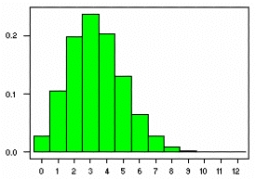# Population variance

In a California community college, 60% of students will transfer to a college in the CSU system. The number of students in a sample who will transfer follows a binomial distribution.

If 8 students are randomly selected, find the population variance σ2 of the random variable.

If 8 students are randomly selected, find the probability 3 or less of these students willp transfer to a college in the CSU system.Did you find an error or inaccuracy? Feel free to write us. Thank you!Tips to related online calculators
Looking for help with calculating arithmetic mean?
Looking for a statistical calculator?
Would you like to compute count of combinations?

#### You need to know the following knowledge to solve this word math problem:

We encourage you to watch this tutorial video on this math problem: